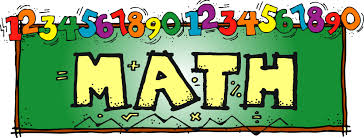# Math InformationMath is a very important part of your child's learning day.  Homework will be assigned nightly to reinforce the skills learned that day.

Homework assignments are graded based on effort and completion of the task. Completing the assigned task with effort earns 2 points.  Completing more of the assignment than asked earns an extra bonus point (3 points total).  Completing less than the assigned task or turning homework in late earns 1 point.  Not turning homework in at all is a 0.  Homework counts for 15% of their overall grade.

Tests make up 50% of the overall grade.  Students are allowed to correct any test below a 75% for a higher grade through January 24th.  After January 24th test corrections are no longer allowed.

Mid-Chapter Checkpoints are considered classwork and make up 35% of the overall grade.  Students are allowed to correct any mid-chapter checkpoint below a 75% for a higher grade through January 24th.  After January 24th test corrections are no longer allowed.

Place Value, Multiplication, and Expressions Whole Numbers and Decimals
Divide Whole Numbers Fractions
Add and Subtract Decimals Rational Numbers
Multiply Decimals Ratios and Rates
Divide Decimals Percents
Add and Subtract Fractions Units of Measure
Multiply Fractions Algebra: Expressions
Divide Fractions Algebra: Equations and Inequalities
Algebra: Patterns and Graphing Algebra: Relationships Between Variables
Convert Units of Measure Area
Geometry and Volume Surface Area and Volume

Data Displays and Measures of Center

Variability and Data Distributions

To access Think Central online and any of the math materials follow the steps below.

1. Go to http://www-k6.thinkcentral.com

2. Select our state, district, and school.